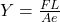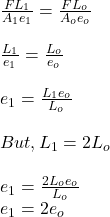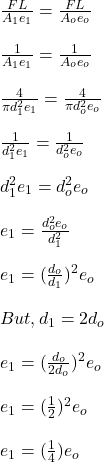## 7. A force stretches a wire by 1 mm. a. A second wire of the same material has the same cross section and twice the length. How far will it

Question

7. A force stretches a wire by 1 mm. a. A second wire of the same material has the same cross section and twice the length. How far will it be stretched by the same force? Explain. b. A third wire of the same material has the same length and twice the diameter as the first. How far will it be stretched by the same force? Explain.

in progress 0
6 months 2021-07-27T08:25:43+00:00 1 Answers 11 views 0

(a) The second wire will be stretched by 2 mm

(b) The third wire will be stretched by 0.25 mm

Explanation:

Tensile stress on every engineering material is given as the ratio of applied force to unit area of the material.

σ = F / A

Tensile strain on every engineering material is given as the ratio of extension of the material to the original length

δ = e / L

The ratio of tensile stress to tensile strain is known as Young’s modulus of the material.Part A

cross sectional area and applied force are the same as the original but the length is doubledThe second wire will be stretched by 2 mm

Part B

a third wire with the same length but twice the diameter of the firste₁ = ¹/₄ x 1 mm = 0.25 mm

The third wire will be stretched by 0.25 mm